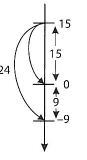Lesson 3 Number lines galore

# Number lines galore

Using the number line with attention to size of number
Overview Resources
Pupils recognise the need for a scale, for which they need at least two reference points given on any number line. They make up a scaled numberline and show on it some subtraction calculations with attention to main markers. They also deal with the temperature scales that are equivalent but have different starting points.

The emphasis here is on intuitive visual sense-making of number size. This is distinct from mental or written calculations, which cause diversions into procedures.
Worksheets 1 and 3 photocopied onto card and cut into strips
Worksheet 2
• Develop the proportional sense of the number line
• Handle number as both a position and a move on the number line.
• Operations on the number line
• Negative numbers
• EPISODE 1
Same length number lines with different scalesPupils sequentially work on three strips with number lines of the same length but different scales. They are to find 15, 8 and 24. On the first strip they address ways of halving an interval and estimating size of the unit. With the second strip they should recognise in their own ways that they cannot have a unique solution, and the need for at least two reference numbers. They should choose a convenient number, different from 30, and label some main markers to place the numbers. The third strip leads to extensions of the number line beyond the visible to place 24.
EPISODE 2
Extending the number line in scaleOn an empty number line pupils mark a scale up to 50 and discuss how to place negative numbers. They place on the line the same numbers 15,8 and 24. Then they show how to find the subtraction sums 24 - 15 and 24 — 8. The aim is to verbalise the use of the 10 markers and visualise the subtraction as an interval in its own right as well as a movement down the number line. Some pupils may handle subtracting the larger number from the smaller, e.g. 8 - 24 and 15 - 24, and explore the answers.
EPISODE 3
Temperature scalesOn two strips, for the F and C temperature ask; How they find temperatures, e.g. 70°F and 24°C? What happens when the temperature rises by 6 degrees and then drops by 16 degrees in each scale?